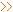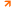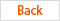Local:IndexServicestechnical support

# Technical Support## 2011-03-17Length
 1 km (km) = 0.621 miles (mile) One meters (m) = 3.281 feet (ft) = 1.094 yards (yd) One centimeter (cm) = 0.394 inches (in) 1 miles (mile) = 1.609 kilometers (km) One feet (ft) = 0.3048 meters (m) 1 inches (in) = 2.54 centimeters (cm) One sea miles (n mile) = 1.852 kilometers (km) One yards (yd) = 0.9144 meters (m) One feet (ft) = 12 inches (in) One yards (yd) = 3 feet (ft) 1 miles (mile) = 5,280 feet (ft) One sea miles (n mile) = 1.1516 miles (mile)Quality
 One t (t) = 1,000 kilograms (kg) = 2205 pounds (lb)= 1.102 short tons (sh.ton) = 0.934 long tons (long.ton) One kilograms (kg) = 2.205 pounds (lb) One short ton (sh.ton) = 0.907 tonnes (t) = 2,000 pounds (1 b) 1 long ton (long.ton) = 1.016 tonnes (t) One pounds (lb) = 0.454 kilograms (kg) One ounces (oz) = 28.350 grams (g)Density
 1 kg / m 3 (kg / m 3) = 0.001 g / cm 3 (g / cm 3) = 0.0624 lb / ft 3 (lb / ft 3) 1 lb / ft 3 (lb / ft 3) = 16.02 kg / m 3 (kg / m 3) 1 lb / 3 inch (lb / in 3) = 27679.9 kg / m 3 (kg / m 3) 1 lb / US gallon (lb / gal) = 119.826 kg / m 3 (kg / m 3) 1 lb / British gallon (lb / gal) = 99.776 kg / m 3 (kg / m 3) 1 lb / (oil) barrels (lb / bbl) = 2.853 kg / m 3 (kg / m 3) 1 Baume density = 140/15.5 ℃, the proportion -130 API = 141.5/15.5 ℃, the proportion -131.5Pressure
 1 MPa (MPa) = 145 lb / 2 inch (psi) = 10.2 kg / cm 2 (kg / cm 2) = 10 bar (bar) = 9.8 atm (at m) 1 lb / 2 inch (psi) = 0.006895 MPa (MPa) = 0.0703 kg / cm 2 (kg / cm 2)= 0.0689 Pakistan (bar) = 0.068 atm (at m) 1 bar (bar) = 0.1 MPa (MPa) = 14.503 lb / 2 inch (psi) = 1.0197 kg / cm 2 (kg / cm 2) = 0.987 atm (at m) 1 atmospheric pressure (at m) = 0.101325 MPa (MPa) = 14.696 lb / 2 inch (psi) = 1.0333 kg / cm 2 (kg / cm 2) = 1.0133 Pakistan (bar)Area
 One square kilometers (km 2) = 100 hectares (ha) = 247.1 Acres (acre) = 0.386 square miles (mile 2) One square meters (m 2) = 10.764 square feet (ft 2) 1 hectares (acre) = 100 square meters (m 2) One hectares (ha) = 10,000 square meters (m 2) = 2.471 acres (acre) 1 square miles (mile 2) = 2.590 square kilometers (km 2) One acres (acre) = 0.4047 hectares (ha) = 40.47 * 10 -3 square kilometers (km 2) = 4,047 square meters (m 2) One square feet (ft 2) = 0.093 square meters (m 2) One square inches (in 2) = 6.452 square centimeters (cm 2) One square yards (yd 2) = 0.8361 square meters (m 2)Volume
 One cubic meters (m 3) = 1,000 liters (liter) = 35.315 cubic feet (ft 3) = 6.290 barrels (bbl) One cubic feet (ft 3) = 0.0283 cubic meters (m 3) = 28.317 liters (l) 1 1,000 cubic feet (mcf) = 28.317 cubic meters (m 3) 1 1 million cubic feet (MMcf) = 2.8317 million cubic meters (m 3) 1 billion cubic feet (bcf) = 2831.7 million cubic meters (m 3) 1 trillion cubic feet (tcf) = 283.17 billion cubic meters (m 3) One cubic inches (in 3) = 16.3871 cubic centimeters (cm 3) One acres feet = 1,234 cubic meters (m 3) 1 barrels (bbl) = 0.159 cubic meters (m 3) = 42 US gallons (gal) 1 US gallon (gal) = 3.785 liters (l) 1 US Qt (qt) = 0.946 liters (l) 1 US pint (pt) = 0.473 liters (l) Kyrgyzstan 1 US ears (gi) = 0.118 liters (l) 1 British gallon (gal) = 4.546 liters (l)Kinematic viscosity
 1 ft 2 / sec (ft 2 / s) = 9.29030 * 10 -2 M 2 / s (m 2 / s) 1 Andean (St) = 10 -4 m 2 / s (m 2 / s) 1% Andean (eSt) = 10 -6 m 2 / s (2 m / s) = 1 mm 2 / s (mm 2 / s)Dynamic viscosity
 1 Mooring (P) = 0.1 Pago seconds (Pa s) 1% Mooring (cP) = 10 -3 Pago seconds (Pa s) 1,000 Caledon seconds / m 2 = 9.80505 Pago seconds (Pa s) One pounds of seconds / ft 2 (1bf s / ft 2)= Pago 47.8803 seconds (Pa s)Power
 1 Newton (N) = 0.225 pounds of (1 bf) = 0.102 000 Caledon (kgf) 1,000 Caledon (kgf) = 9.81 Newton (N) One pounds of (1 bf) = 4.45 Newton (N) 1 dyne (dyn) = 10 -5 Newton (N)Temperature
 K (Kelvin degrees) = 5 / 9 (℉ +459.67) K=℃+273.15 n℉=[(n-32)*5/9]℃ N ° C (degrees Celsius) = (5 / 9 n +32) ° F 1 ° F (degrees Fahrenheit) = 5 / 9 ° C (the temperature difference)Heat transfer coefficient
 1 kcal (m 2:00 ° C) [kcal / (M 2 h ℃)] = 1.6279 watts / (m 2 Kelvin) [W (m 2 K)] 1 British thermal units / (ft 2:00 ° F) [Btu / (ft 2 h ℉)] = 5.67826 watts / (m 2 Kelvin) [W (m 2 K)] 1 m 2:00 ℃ / kilocalories (m 2 h ℃ / kcal) = 0.86000 m 2 Kelvin / watt (m 2 K / W) 1 kcal (m 2:00) (kcal / m 2 h) = 1.16279 watts / m 2 (W / m 2)Thermal conductivity
 1 kcal (m 2:00 ° C) [kcal / (m 2 h ° C)] = 1.16279 watts / (meter Kelvin) [W (m K)] 1 British thermal units / (ft 2:00 ℉) [Btu / (ft 2 h ℉)] = 1.7303 watts / (meter Kelvin) [W (m K)]hematocrit heat
 1 kcal / (kg ° C) [kcal / (kg ° C)] = 1 British thermal units / (pounds ℉) [Btu / (lb ℉)] = 4186.8 joules / (kg Kelvin) [J / (kg K)]thermal power
 1 Joule = 0.10204 000克米= 2.778 * 10 -7 kWh = 3.777 * 10 -7 metric horsepower hour = 3.723 * 10 -7 Imperial horsepower hour = 2.389 * 10 -4 kilocalories = 9.48 * 10 -4 British thermal units 1 Card (cal) = 4.1868 joules (J) 1 British thermal units (Btu) = 1055.06 joules (J) 1,000 Caledon meters (kgf m) = 9.80665 joules (J) 1 ft pounds of (ft 1bt) = 1.35582 joules (J) 1 m-horsepower hour (hp h) = 2.64779 * 10 6 joules (J) 1 Imperial horsepower hour (UKHp h) = 2.68452 * 10 6 joules (J) 1 kilowatt hours (kw h) = 3.6 * 10 6 joules (J) 1 kcal = 4186.75 joules (J)Power
 1,000 Caledon m / s (kgf m / s) = 9.80665 watts (W) 1 m-horsepower (hp) = 735.499 watts (W) 1 Card / sec (cal / s) = 4.1868 watts (W) 1 British thermal units / pm (Btu / h) = 0.293071 watts (W)Speed
 1 ft / sec (ft / s) = 0.3048 meters / second (m / s) 1 miles / pm (mile / h) = 0.44704 meters / second (m / s)Penetration
 1 Darcy = 1000 cents Darcy One square centimeters (cm 2) = 9.81 * 10 7 DarcyThe technology data for  Heat transfer coeff,